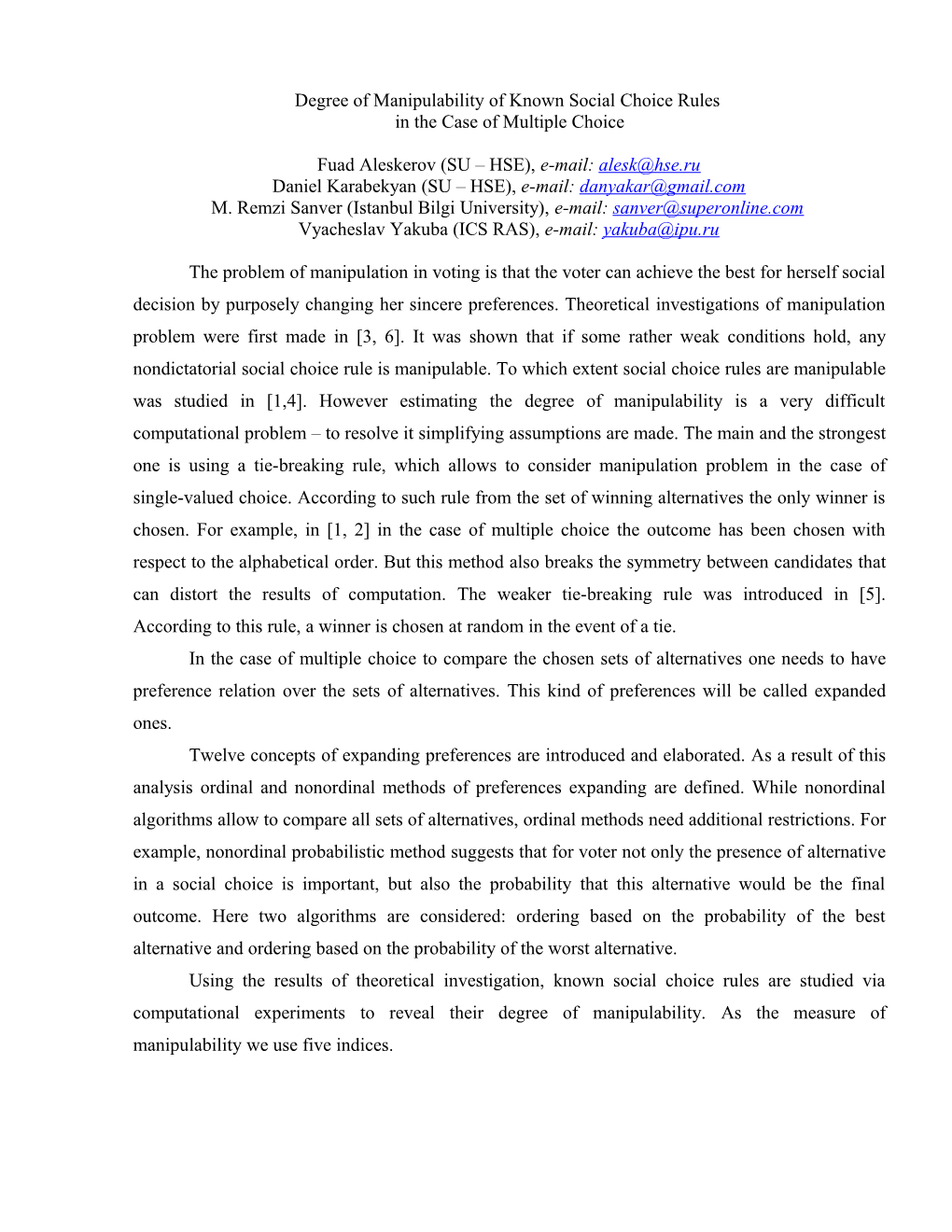# Degree of Manipulability of Known Social Choice Rules in the Case of Multiple ChoiceDegree of Manipulability of Known Social Choice Rules

in the Case of Multiple Choice

Fuad Aleskerov (SU – HSE), e-mail:

Daniel Karabekyan (SU – HSE), e-mail:

M. Remzi Sanver (IstanbulBilgiUniversity), e-mail:

## Vyacheslav Yakuba (ICS RAS), e-mail:

The problem of manipulation in voting is that the voter can achieve the best for herself social decision by purposely changing her sincere preferences. Theoretical investigations of manipulation problem were first made in [3, 6]. It was shown that if some rather weak conditions hold, any nondictatorial social choice rule is manipulable. To which extent social choice rules are manipulable was studied in [1,4]. However estimating the degree of manipulability is a very difficult computational problem – to resolve it simplifying assumptions are made. The main and the strongest one is using a tie-breaking rule, which allows to consider manipulation problem in the case of single-valued choice. According to such rule from the set of winning alternatives the only winner is chosen. For example, in [1, 2] in the case of multiple choice the outcome has been chosen with respect to the alphabetical order. But this method also breaks the symmetry between candidates that can distort the results of computation. The weaker tie-breaking rule was introduced in . According to this rule, a winner is chosen at random in the event of a tie.

In the case of multiple choice to compare the chosen sets of alternatives one needs to have preference relation over the sets of alternatives. This kind of preferences will be called expanded ones.

Twelve concepts of expanding preferences are introduced and elaborated. As a result of this analysis ordinal and nonordinal methods of preferences expanding are defined. While nonordinal algorithms allow to compare all sets of alternatives, ordinal methods need additional restrictions. For example, nonordinal probabilistic method suggests that for voter not only the presence of alternative in a social choice is important, but also the probability that this alternative would be the final outcome. Here two algorithms are considered: ordering based on the probability of the best alternative and ordering based on the probability of the worst alternative.

Using the results of theoretical investigation, known social choice rules are studied via computational experiments to reveal their degree of manipulability. As the measure of manipulability we use five indices.

The calculation of indices is performed for up to m = 5 alternatives. For small number of voters n all possible profiles are checked for manipulability and respective indices are evaluated. For greater number of voters the statistical scheme is used.

For each profile under consideration in both exhaustive and statistical schemes, all m!-1 manipulating orderings for each voter are generated and the respective choice sets of manipulating profiles are compared with the choice of the original profile, using all introduced methods of the preference extensions.

The results for known social choice rules and more alternatives will be reported.

### References

1. Aleskerov F, Kurbanov E (1999) «Degree of manipulability of social choice procedures», in: Alkan et al. (eds.) Current Trends in Economics. Springer, BerlinHeidelbergNew York
2. Favardin P, Lepelley D (2006) «Some further results on the manipulability of social choice rules», Social Choice and Welfare Volume 26: 485-509
3. Gibbard A (1973) «Manipulation of voting schemes», Econometrica Volume 41: 587-601
4. Kelly J (1993) «Almost all social choice rules are highly manipulable, but few aren’t», Social Choice and Welfare Volume 10: 161-175
5. Pritchard G, Wilson M (2005) «Exact results on manipulability of positional voting rules», CDMTCS Research Report Series
6. Satterthwaite M (1975) «Strategy-proofness and Arrow.s conditions: existence and correspondence theorems for voting procedures and social welfare functions», Journal of Economic Theory Volume 10: 187-217